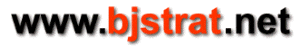```Expected value (EV) of a given player hand in blackjack depends upon the probability of winning
and the probability of losing for a given strategy.

For a given player hand in blackjack versus a given dealer up card, player can expect to win, lose,
and tie (push) some percentage of the time depending upon strategy.
Let w(strat) = probability of winning the hand for a given strategy
Let l(strat) = probability of losing the hand for a given strategy
Let t(strat) = probability of tying (pushing) the hand for a given strategy
then w(strat)+l(strat)+t(strat) = 1 (sum of probabilities of winning, losing, and tying = 1 = 100%)

If strategy is stand, EV(stand) = w(stand) - l(stand)
If strategy is hit, EV(hit) = w(hit) - l(hit)
If strategy is double, EV(double) = 2*w(double) - 2*l(double)
If strategy is split, 2 or more hands occur before dealer draws and EV(split) = sum of EVs of each hand
If strategy is late surrender (up card is T or A), EV(LS) = -(prob dealer BJ) - .5*(1 - prob dealer BJ)
If strategy is early surrender (up card is T or A), EV(ES) = -.5
If strategy is surrender (up card is 2 through 9), EV(surrender) = -.5

With simulation values for w(strat), l(strat), and t(strat) are arrived at by recording a large number
of trials that play each strategy (drawing dealer cards if necessary) until it wins, loses, or ties.

With combinatorial analysis w(strat) and l(strat) are computed by going through every possibility. In
order to compute EV the probability of tying is not necessary but it could optionally be computed. (The
probability of tying is necessary in order to compute variance/standard deviation.)

****************************************************************************************************************

The EV of a hand is expressed relative to the amount bet before the hand is dealt:
For strategies of stand or hit the most a player can win or lose is the original bet
An EV of -1 = -100% means player can expect to lose an amount equal to the original bet in the long run
An EV of 0 = 0% means a player can expect to break even in the long run
An EV of +1 = +100% means player can expect to win an amount equal to the original bet in the long run

On doubles player adds an amount equal to the original bet and can win or lose up to twice the original bet
An EV of -2 = -200% means player can expect to lose an amount equal to twice the original bet in the long run
An EV of -1 = -100% means player can expect to lose an amount equal to the original bet in the long run
An EV of 0 = 0% means a player can expect to break even in the long run
An EV of +1 = +100% means player can expect to win an amount equal to the original bet in the long run
An EV of +2 = +200% means player can expect to win an amount equal to twice the original bet in the long run

On splits player adds an amount equal to the original bet for each new hand. Depending upon rules player may
also be able to double a new split hand and would add another amount equal to the original bet in order to
double. If rules are to allow 3 splits then player may have up to 4 split hands, each of which might be doubled.
Therefore on splits player can win or lose an amount up to 8 times the original bet.
An EV of -8 = -800% means player can expect to lose an amount equal to 8 times original bet in the long run
An EV of -7 = -700% means player can expect to lose an amount equal to 7 times original bet in the long run
An EV of -6 = -600% means player can expect to lose an amount equal to 6 times original bet in the long run
An EV of -5 = -500% means player can expect to lose an amount equal to 5 times original bet in the long run
An EV of -4 = -400% means player can expect to lose an amount equal to 4 times original bet in the long run
An EV of -3 = -300% means player can expect to lose an amount equal to 3 times original bet in the long run
An EV of -2 = -200% means player can expect to lose an amount equal to 2 times original bet in the long run
An EV of -1 = -100% means player can expect to lose an amount equal to the original bet in the long run
An EV of 0 = 0% means player can expect to break even in the long run
An EV of +1 = +100% means player can expect to win an amount equal to the original bet in the long run
An EV of +2 = +200% means player can expect to win an amount equal to 2 times original bet in the long run
An EV of +3 = +300% means player can expect to win an amount equal to 3 times original bet in the long run
An EV of +4 = +400% means player can expect to win an amount equal to 4 times original bet in the long run
An EV of +5 = +500% means player can expect to win an amount equal to 5 times original bet in the long run
An EV of +6 = +600% means player can expect to win an amount equal to 6 times original bet in the long run
An EV of +7 = +700% means player can expect to win an amount equal to 7 times original bet in the long run
An EV of +8 = +800% means player can expect to win an amount equal to 8 times original bet in the long run

****************************************************************************************************************
```